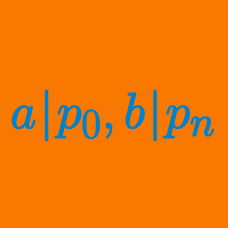Algebra

Rational Root Theorem: Level 2 Challenges

How many rational roots does ${ x }^{ 1000 }-{ x }^{ 500 }+{ x }^{ 100 }+x+1=0$ have?

Find the sum of all real roots of

$\ 27x^3+21x+8 = 0$

Given that $p$ and $q$ are both prime, which of the following answer choices is true about the equation $px^{ 2 }-qx+q=0?$

The polynomial $x^3 - 3x^2 + 12x + 16$ has one real root $r$ and two complex roots $w$ and $z.$ What is the value of $r+ \log_2 \left(w^6 + z^6 \right)$?

Two of the roots of the equation $ax^3 + bx^2 + cx + d = 0$ are $2 - \sqrt{3}$ and $5$, where $a,b,c,d$ are rational numbers. If the third one is $m + \sqrt{n}$ for some integers $m$ and $n$, find $m + n$

×